• 后缀表达式求值,练习堆栈，算法。大家需要可以参考下
• 算法之用后缀表达式求值思路中序表达式转后序表达式之前博客写过。数据结构是栈当是数字时，压入栈。当是操作符时将栈元素弹出两个计算结果，将结果压入栈。最后返回栈顶元素。栈顶元素即为求值结果。实现import ...
算法之用后缀表达式求值思路中序表达式转后序表达式之前博客写过。数据结构是栈当是数字时，压入栈。当是操作符时将栈元素弹出两个计算结果，将结果压入栈。最后返回栈顶元素。栈顶元素即为求值结果。实现import java.util.List;import java.util.Scanner;import java.util.Stack;public class EvaluatePostfix {public static void main(String[] args) {Scanner scan = new Scanner(System.in);float result = 0;if(scan.hasNextLine()){String str = scan.nextLine();result = evaluatePostfix(str);}System.out.println(result);}public static Float evaluatePostfix(String input){Stack stack = new Stack<>();String[] data = input.split(" ");for(int i = 0 ;i < data.length ; i++){if( data[i].equals("+") || data[i].equals("-") || data[i].equals("*") || data[i].equals("/")){float a = stack.pop();float b = stack.pop();if(data[i].equals("+")){stack.push(b+a);}else if(data[i].equals("-")){stack.push(b-a);}else if(data[i].equals("*")){stack.push(b*a);}else if(data[i].equals("/")){stack.push(b/a);}}else if(data[i].equals("")){continue;}else{stack.push(Float.valueOf(data[i]));}}return stack.pop();}}运行结果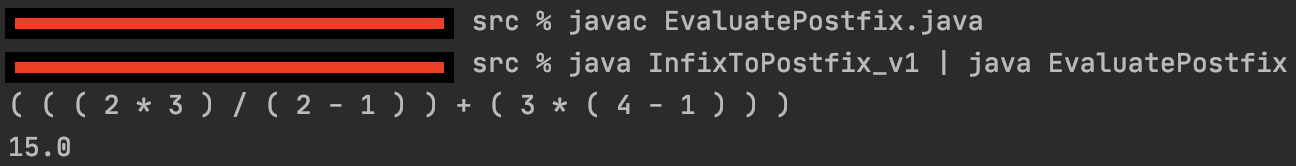本文地址：https://blog.csdn.net/qq_40676509/article/details/110231259希望与广大网友互动？？点此进行留言吧！
展开全文• 表达式求值中缀表达式求值中缀表达式转后缀表达式后缀表达式求值（逆波兰表达式求值）前缀表达式（波兰表达式） 首先，大家可能不知道前缀表达式和中缀表达式，后缀表达式是什么，其有什么区别呢。我先简单介绍一下...
表达式求值中缀表达式求值中缀表达式转后缀表达式后缀表达式求值（逆波兰表达式求值）前缀表达式（波兰表达式）
首先，大家可能不知道前缀表达式和中缀表达式，后缀表达式是什么，其有什么区别呢。我先简单介绍一下：

前缀表达式是一种没有括号的算术表达式，与中缀表达式不同的是，其将运算符写在前面，操作数写在后面。为纪念其发明者波兰数学家Jan Lukasiewicz，前缀表达式也称为“波兰式”。例如，- 1 + 2 3，它等价于1-(2+3)

中缀表达式及我们正常的式子eg:1-(2+3)

后缀表达式： 逆波兰式（Reverse Polish notation，RPN，或逆波兰记法），也叫后缀表达式（将运算符写在操作数之后）eg:1-(2+3)      1 2 3+ -

中缀表达式求值
中缀表达式即我们正常的表达式，我们先讲一下其求值规则：

1.通过一个index值（索引），来完成我们的表达式
2.如果我们发现是一个数字，就直接入数栈
3.如果我们发现是一个符号，分一下情况
3.1.如果发现当前的符号栈为空，就直接入栈
3.2.如果符号栈中有操作符，就进行比较，如果当前操作符的优先级小于或者等于栈中的操作符，就需要从数栈中pop出一个符号，进行运算，将得到的结果入数栈，然后将当前的操作符入符号栈，如果当前操作符的优先级大于栈中的操作符，就直接入符号栈。如果有括号 先将左括号入栈 碰到右括号的时候，将符号栈中栈顶元素出栈，数字栈中两次出栈顶元素，进行运算，将运算的结果插入数字栈中， 直到为右括号便停止该循坏 并移除符号栈中的左括号
4.当表达式扫描完毕，就顺序的从数栈和符号栈中pop出相应的数和符号，并进行运算。
5.最后在数栈只有一个数字，就是表达式的结果

首先我们来模拟一下：
比如：(3+4)*5-2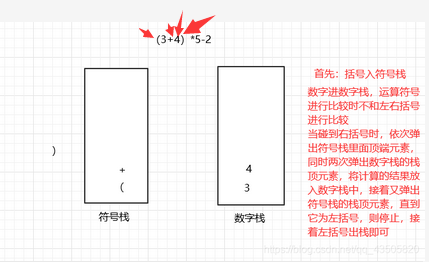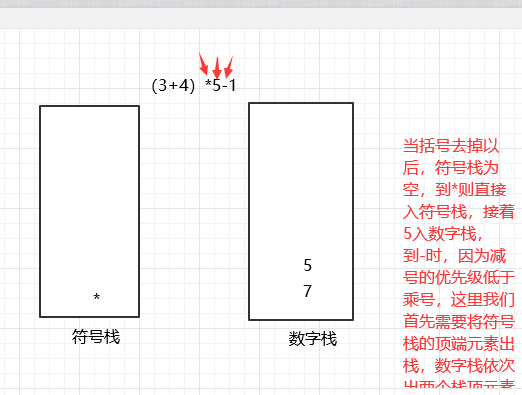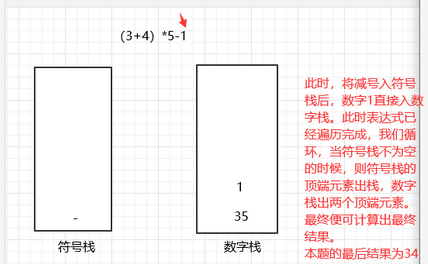至此步骤结束，大家可以自己多模拟几遍。
代码：
首先我模拟了一个栈，里面添加了关于优先级的判断和是否为运算符号的判断，大家可以以只看这两种方法即可。
class ArrayStack{
private int maxSize;//栈的大小
private int[] stack;//数组，存放数据
private int top=-1;//top表示栈顶，初始化为-1
public ArrayStack(int maxSize){
this.maxSize=maxSize;
stack=new int[maxSize];
}
//栈满
public boolean isFull(){
return top==maxSize-1;
}
//栈空
public boolean isEmpty(){
return top==-1;
}
//入栈
public void push(int data){
if(isFull()){
System.out.println("栈满,插入失败");
return;
}
stack[++top]=data;
}
//出栈
public int pop(){
if(isEmpty()){
System.out.println("栈空，无元素");
return -1;
}
int data=stack[top];
top--;
return data;
}
//获取顶端元素
public int peek(){
return stack[top];
}
//显示栈的情况(遍历栈，从上往下)
public void list(){
if(isEmpty()){
System.out.println("栈空");
return;
}
for (int i = top; i >=0; i--) {
System.out.print(stack[i]+" ");
}
}

//用于判断优先级  在进行表达式运算时需要用到
public int priority(int oper){
if(oper=='*'||oper=='/'){
return 1;//优先级最高
}
else if(oper=='+'||oper=='-'){
return 0;
}
else return -1;
}
//判断是不是一个运算符
public boolean isOper(char val){
return val=='+'||val=='-'||val=='*'||val=='/'||val=='('||val==')';
}
//计算方法
public int cal(int num1,int num2,int oper){
int res=0;
switch (oper){
case '+':
res=num1+num2;
break;
case '-':
res=num2-num1;
break;
case '*':
res=num1*num2;
break;
case '/':
res=num2/num1;
break;
default:break;
}
return res;
}
}

public class ComputerDemo {
public static void compute(String expression){
ArrayStack numStack=new ArrayStack(10);
ArrayStack operStack=new ArrayStack(10);
int index=0;
int num1=0;//表示输出数字的栈顶元素
int num2=0;//表示地如此输出数字栈的栈顶元素
int oper=0;//运算符号  符号栈的栈顶元素
int res=0;//计算结果
char ch=' ';//将每次扫描得到的char保存到ch
String keepNum="";//防止不止一个数字 为多位数
//开始while循环的扫描expression
while (index!=expression.length()) {
//依次得到expression的每一个字符
ch = expression.substring(index, index + 1).charAt(0);
//判断ch是什么，然后做相应的处理
if(operStack.isOper(ch)){
/**
*  3.1.如果发现当前的符号栈为空，就直接入栈
*  3.2.如果符号栈中有操作符，就进行比较，如果当前操作符的
*    优先级小于或者等于栈中的操作符，就需要从数栈中pop出一个符号，
*    进行运算，将得到的结果入数栈，然后将当前的操作符入符号栈，如果当前操作符
*    的优先级大于栈中的操作符，就直接入符号栈
*
*    如果有括号 先将左括号入栈 碰到右括号的时候，将符号栈中栈顶元素出栈，数字栈中两次出栈顶元素，进行运算，
*    将运算的结果插入数字栈中，直到为右括号便停止该循坏 并移除符号栈中的左括号
*/
if(ch=='('){
operStack.push(ch);
}
else if(ch==')'){
//获取符号并进行运算 进行消括号操作
while (operStack.peek()!='(') {
oper=operStack.pop();
num1 = numStack.pop();
num2 = numStack.pop();
res = numStack.cal(num1, num2, oper);
//把运算结果入数栈
numStack.push(res);
}
operStack.pop();//去掉左括号
}
else if (!operStack.isEmpty()) {
//判断当前符号栈是否为空
if (operStack.priority(ch) <= operStack.priority(operStack.peek())) {
num1 = numStack.pop();
num2 = numStack.pop();
oper = operStack.pop();
res = numStack.cal(num1, num2, oper);
//把运算结果入数栈
numStack.push(res);
//然后将当前的操作符入符号栈
operStack.push(ch);
}
else {
//如果当前的操作符的优先级大于栈中的操作符，就直接入符号栈
operStack.push(ch);
}
}else {
//如果为空则直接入符号栈
operStack.push(ch);
}
}
else {//如果是数，则直接入数栈
keepNum+=ch;
//如果ch已经是最后一位，则直接入栈
if(index==expression.length()-1){
numStack.push(Integer.parseInt(keepNum));
}else {
//判断下一位是不是数字，如果是数字，就继续扫描，如果是运算符则入栈
//注意看后一位，不是index++
if(operStack.isOper(expression.substring(index+1,index+2).charAt(0))){
//如果后一位是运算符，则入栈keepNum="1" "123"
numStack.push(Integer.parseInt(keepNum));
// keepNum清空
keepNum="";
}
}
}
//让index+1,并判断是否扫描到expression最后
index++;
}
//当表达式扫描完毕，就顺序的从数栈和符号栈中pop出相应的数和符号，并运行
while (!operStack.isEmpty()){
num1=numStack.pop();
num2=numStack.pop();
oper=operStack.pop();
res=numStack.cal(num1,num2,oper);
numStack.push(res);
}
//将数栈的最后数，pop出，就是结果
int res2=numStack.pop();
System.out.printf("表达式%s = %d",expression,res2);
}
public static void main(String[] args) {
String expression="(2-4)+3*6";
compute(expression);
}
}


中缀表达式转后缀表达式
大家可能会想：既然中缀表达式可以计算结果，我们为什么还要学习后缀表达呢？ 而且后缀表达式看起来不容易理解：
后缀表达式是计算机通常用的方法，因为它不用考虑优先级，可以直接进行出栈运算即可。
所以我们先学习一下如何使中缀表达式转化为后缀表式。
需要了解其法则：

中缀转后缀表达式

1.初始化两个栈，运算栈S1和存储中键结果的栈s2
2.从左至右扫描中缀表达式
3.遇到操作数时，将其压s2
4.遇到运算符时，比较其与s1栈顶运算符的优先级
4.1） 如果s1为空，或栈顶运算符为左括号，则直接将此运算符入栈
4.2)否则，如果优先级比栈顶运算符的高，也将运算符压入s1.
4.3)否则，将s1栈顶的运算符弹出并压入到s2中，再次转到4.1与s1中新的栈顶运算符相比较
5.遇到括号时：
5.1）如果是左括号，则直接入栈
5.2）如果是右括号，则依次弹出s1栈顶的运算符，并压入s2，直到遇到左括号为止，此时将这一对括号丢弃
6)重复步骤2-5 直到表达式的最右边
7）将s1剩余的运算符依次弹出并压入s2
8）依次弹出s2中的元素并输出，结果的逆序即为中缀表达式对应的后缀表达式

我们来模拟一下其转化过程：
eg:(3+4)*5-2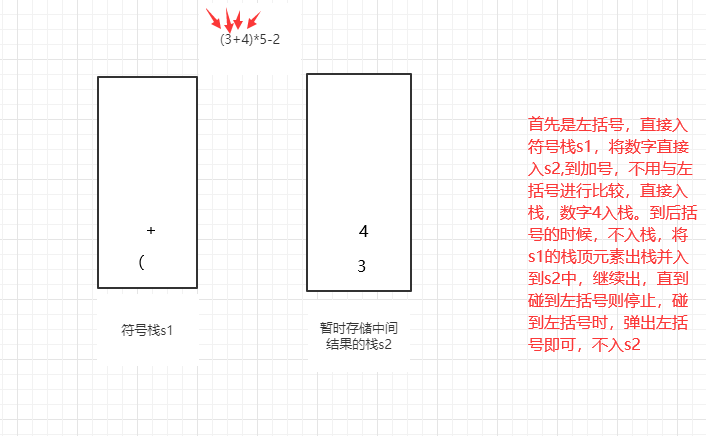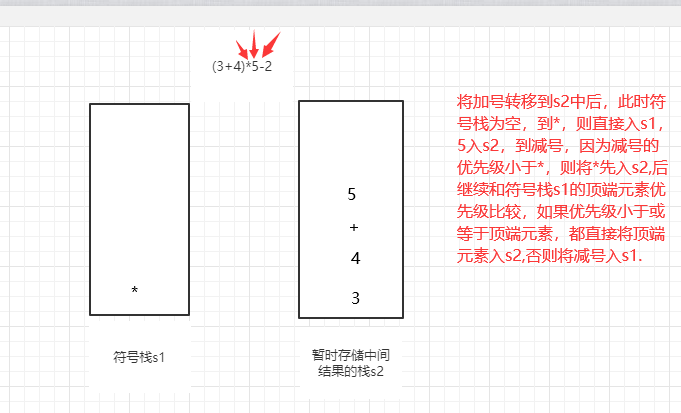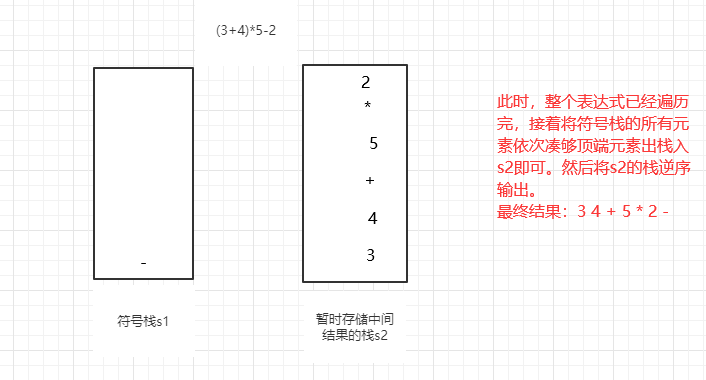需要注意的是，这里进行模拟的时候，采用的是两个栈，但是s2只有插入没有输出的过程，到最后运算完后，还需要将s2栈逆序输出，反而增加了复杂度，因此我们s2可以采用队列，list集合等方便输出。
代码：
首先将中缀表达式转为List集合，方便取元素：
//方法：将中缀表达式转成对应的List
public static List<String> toInfixExpressionList(String s){
//定义一个List，存放中缀表达式 对应的内容
List<String> ls=new ArrayList<>();
int i=0;
String str;//对多位数进行处理
char c=' ';
do {
//如果c是一个非数字，需要加入ls
if((c=s.charAt(i))<'0'||(c=s.charAt(i))>'9'){
ls.add(""+c);
i++;
}
else {
//如果是数字则需要考虑多位数
str = "";//先将str置为“”
while (i<s.length()&&(c=s.charAt(i))>='0'&&(c=s.charAt(i))<='9'){
str+=c;//拼接
i++;
}
ls.add(str);
}
}while (i<s.length());
return ls;
}

其次将表达式转为后缀表达式：
public  static List<String> reverse(List<String> ls){
//定义两个栈
Stack<String> s1=new Stack<>();//符号栈
//说明：因为s2这个栈，在整个转换过程中，没有pop操作，而且后面还需要逆序输出
//比较麻烦，则我们此处使用List<String> s2;
List<String> s2=new ArrayList<>();//储存中间结果的List2
//遍历ls
for(String item:ls){
//如果是数字，则直接放入s2
if(item.matches("\\d+")){
s2.add(item);
}
//左括号直接入栈
else if(item.equals("(")){
s1.push(item);
}//如果是右括号，则依次弹出s1栈顶的运算符，并压入s2,直到遇到左括号为止
else  if(item.equals(")")){
while (!s1.peek().equals("(")){
s2.add(s1.pop());
}
s1.pop();//将（弹出s1栈，消除小括号
}else {
//当item的优先级小于或者等于s1栈顶优先级，先将s1栈顶的运算符弹出并加入到s2中，再次转到4.1与s1中新的
//栈顶运算符相比较
while (s1.size()!=0&&operation.getValue(s1.peek())>=operation.getValue(item)){
s2.add(s1.pop());
}
//还需要将item入栈
s1.push(item);
}
}
while (s1.size()!=0){
s2.add(s1.pop());
}
return s2;//注意因为存放到List中，因此按顺序暑促的就是对应的后缀表达式对应的List
}

此处s1栈，涉及到优先级的比较：
//编写一个类 可以返回运算符对那个的优先级
class operation{
private static int add=1;
private static int sub=1;
private static int mul=2;
private static int div=2;
//写方法 返回其对应的优先级数字
public static  int getValue(String operation){
int res=0;
switch (operation){
case "+":res=add;break;
case "-":res=sub;break;
case "*":res=mul;break;
case "/":res=div;break;
default:
break;
}
return res;
}
}


便可以完成中缀转后缀。
后缀表达式求值（逆波兰表达式求值）
后缀表达式求值比较简单，不需要进行优先级判断，直接出栈计算即可。

从左至右扫描表达式，遇到数字时，将数字压入堆栈

遇到运算符号时，弹出栈顶的两个数，用运算符对他们做相应的计算（次顶元素和栈顶元素），并将结果入栈；
重复上述过程直至表达式最右边，最后运算得出的值即为表达式的结果
eg:(3+4)*5-2  其对应的后缀表达式：3 4 + 5 * 2 -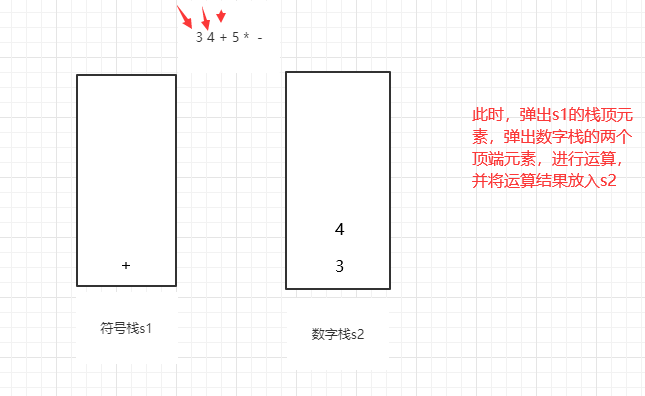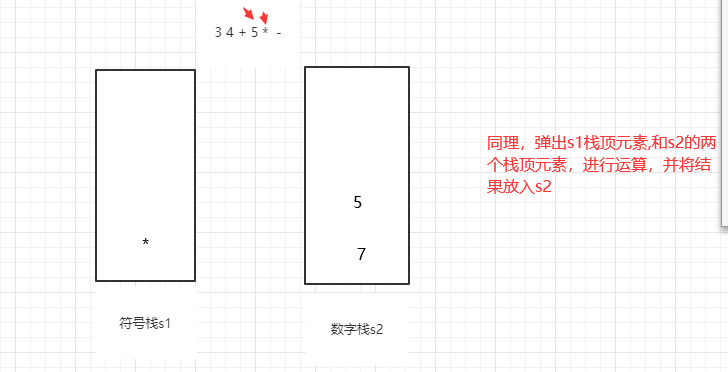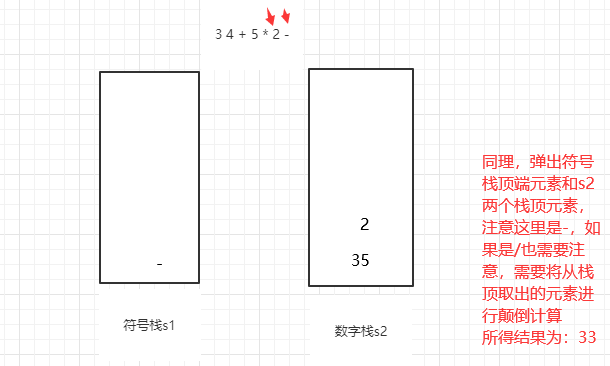代码：
 //完成对逆波兰表达式的计算
public static int compute(List<String> list){
Stack<String> stack=new Stack<>();
for(String item:list){
//使用正则表达式来取数
if(item.matches("\\d+")){
stack.push(item);
}
else {
//pop出两个数 并进行运算  再将结果入栈
int num1=Integer.valueOf(stack.pop());
int num2=Integer.valueOf(stack.pop());
int res=0;
if(item.equals("+")){
res=num1+num2;
}
else if(item.equals("-")){
res=num2-num1;
}
else if(item.equals("*")){
res=num1*num2;
}
else if(item.equals("/")){
res=num2/num1;
}else {
throw new RuntimeException("运算符号有误");
}
stack.push(String.valueOf(res));
}
}
return Integer.valueOf(stack.pop());
}
//将一个逆波兰表达式，依次将数据和运算符放入到Arraylist中
public static List<String> getListString(String suffixExpression){
//将sufferExpression分割
String[] split=suffixExpression.split(" ");
List<String> list=new ArrayList<>();
for(String str:split){
list.add(str);
}
return list;
}

前缀表达式（波兰表达式）
前缀表达式与后缀表达式正好相反，懂了后缀表达式，便可以轻松写出前缀表达式
需要注意：前缀表达式 符号在前，数字在后，我们进行运算时，需要从右至左进行运算，其余步骤一样。
例如，- 1 + 2 3，它等价于1-(2+3)。大家举一反三，便可以自己求出，本文不再赘述。
有什么问题欢迎大家前来提问。


展开全文java 数据结构 算法...